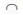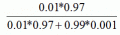# Chapter 7: Probability

Quizzes are available to test your understanding of the key concepts covered in each chapter. Click on the quiz below to get started.

1. The probability density function tells us about:

1. The value of a score given a certain probability
2. The relationship between the outcome of a random variable and its probability
3. The probability of obtaining a score bigger than a set value
4. The shape of the distribution of a non-normal variable

The correct answer is B. The density function once we have transformed raw scores into z scores, it allows us to find the probability for a score bigger or smaller than a particular value.

2. Given the sample space is given by E = {1, 2, 3, 4, 5, 6}, A = {1, 5}, B = {3, 5, 6} and C = {2, 4, 6} and D ={1, 3, 5}. What is the event of:

1.B
2. A  U B
3.D
4. B U D

The correct answer is Shorts answers: A. {5}; b) {1, 3, 5, 6}; c) None; d) {1, 3, 5, 6}

3. In a physics exam, a student has only prepared 15 out of the 25 topics of the questionnaire. The test consists of answering about two topics randomly chosen. What is the probability that the two chosen topics are the ones the student has prepared?

1. 0.25
2. 0.45
3. 0.35
4. 0.02

The correct answer is C. The probability is B1B2. The events are dependent, so the probability is equal to p(B1) * p(B1/ B1) = 15/25 * 14/24 = 0.35

4. You have been tasked to print a survey, but the printer displays a message stating that for every 1,000 copies, 12 are badly printed. Find the solution for the following:

1. The probability that a survey is badly printed
2. The probability that a survey is correctly printed
3. The probability that a survey is both

The correct answer is Short answers: a) p(badly printed) = 12/1000 = 0.012; b) p(correctly printed) = 1 – p(badly printed) = 1 – 0.012 = 0.988; c) the events are independent and cannot happen at the same time.

5. In a large urban city, the probability of their citizens having cancer is 0.01. We have randomly chosen an individual and we have performed an X-ray to detect cancer. The probability of the X-ray machine detecting cancer is 0.97 if it really has cancer, and 0.001 if it doesn’t. What can we conclude about the probability of the person chosen?

1. 0.91 chance of correctly detecting cancer
2. 0.15 chances of correctly detecting cancer
3. 0.85 chances of correctly detecting cancer
4. 0.05 chances of incorrectly detecting cancer

The correct answer is A. The probability to calculate is p(having cancer/have been detected) == 0.907 or 0.91.